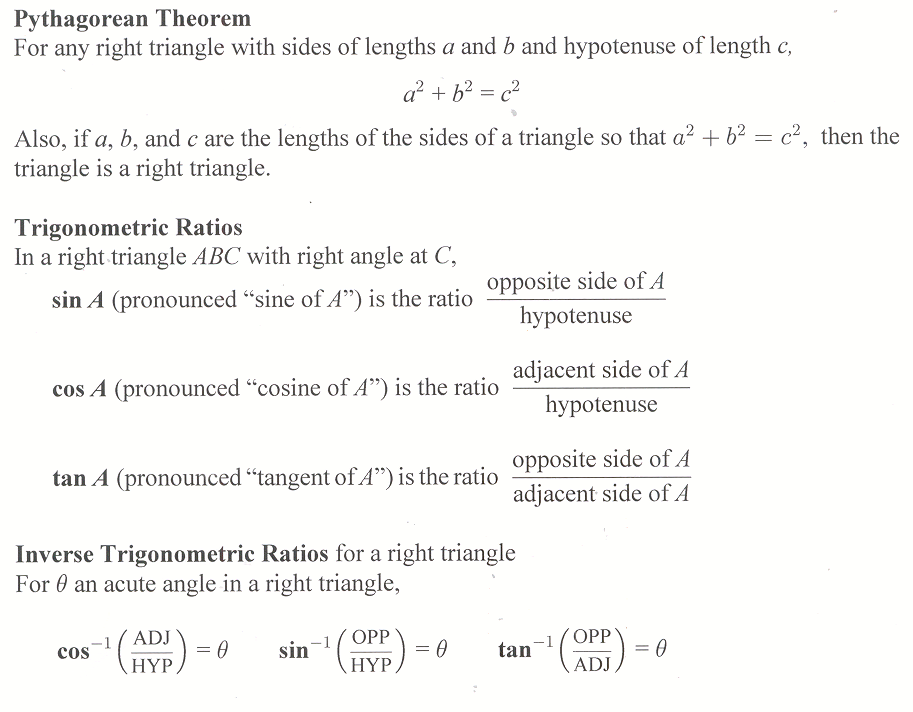## Homework Hints 7-5

### Note: Homework Hints are given only for the Level 1 and Level 2 problems.

However, as you go through the book be sure you look at all the examples in the text. If you need hints for the Level 3 … See the whole entry

## 7.5 Outline

1. Pythagorean theorem
2. Trigonometric ratios
1. sine
2. cosine
3. tangent
3. Inverse trigonometric ratios
1. calculator calculating
2. inverse trigonometric ratios
1. inverse tangent
2. inverse cosine
3. inverse sine
4. Applications
1. angle of elevation
2. angle of depression

## 7.5 Essential IdeasSee the whole entry

## Individual Research Projects Section 5.5

### Project 5.8

You may have seen a geoboard, which is simply a board with pegs. Two simple geoboards with the pegs labeled A, B, C, ….

Distance on a Geoboard

Suppose that the horizontal and vertical distance between the pegs … See the whole entry

## Homework Hints 5-5

### Note: Homework Hints are given only for the Level 1 and Level 2 problems.

However, as you go through the book be sure you look at all the examples in the text. If you need hints for the Level 3 … See the whole entry

## 5.5 Outline

1. Pythagorean theorem
1. perfect squares
2. square numbers
3. legs/hypotenuse (There is a nice little graphic showing the meaning of the Pythagorean theorem below)
2. Square roots
1. definition
2. positive square root of n
3. irrational numbers
4. the number pi
3. Operations with square roots
See the whole entry